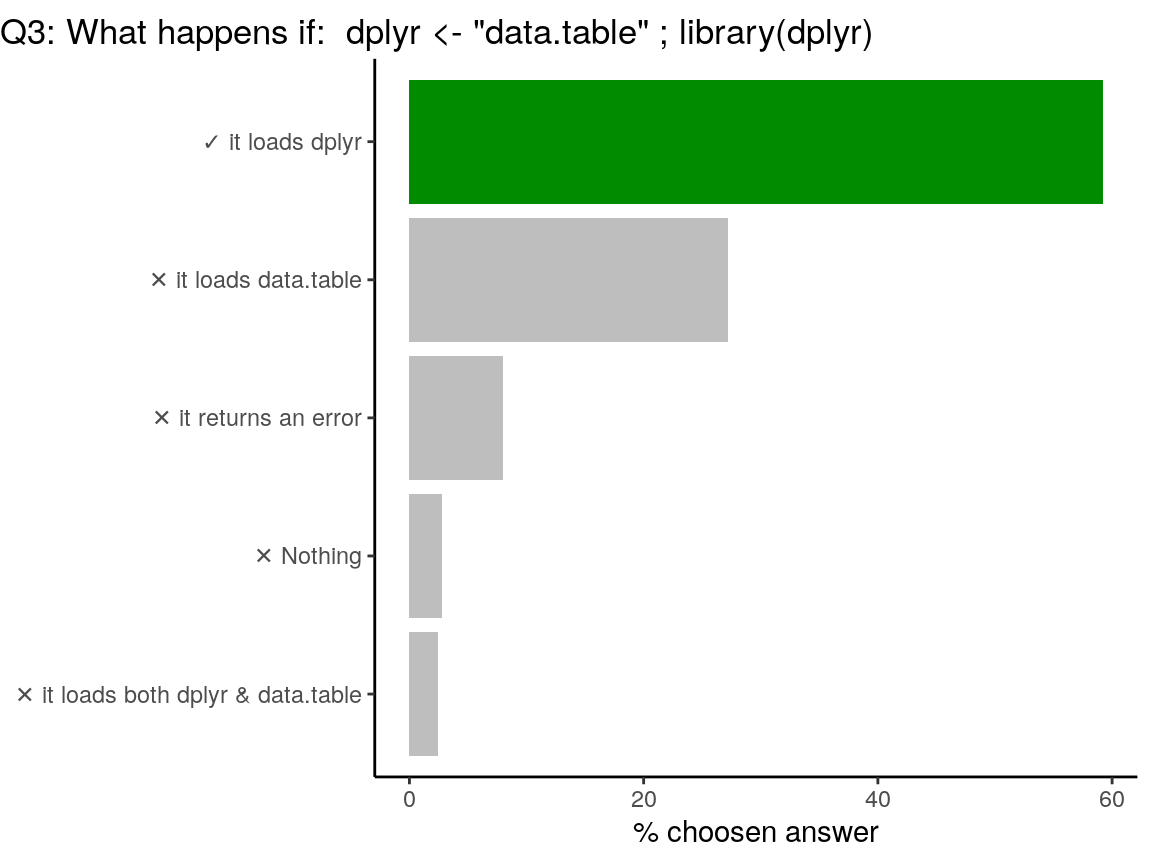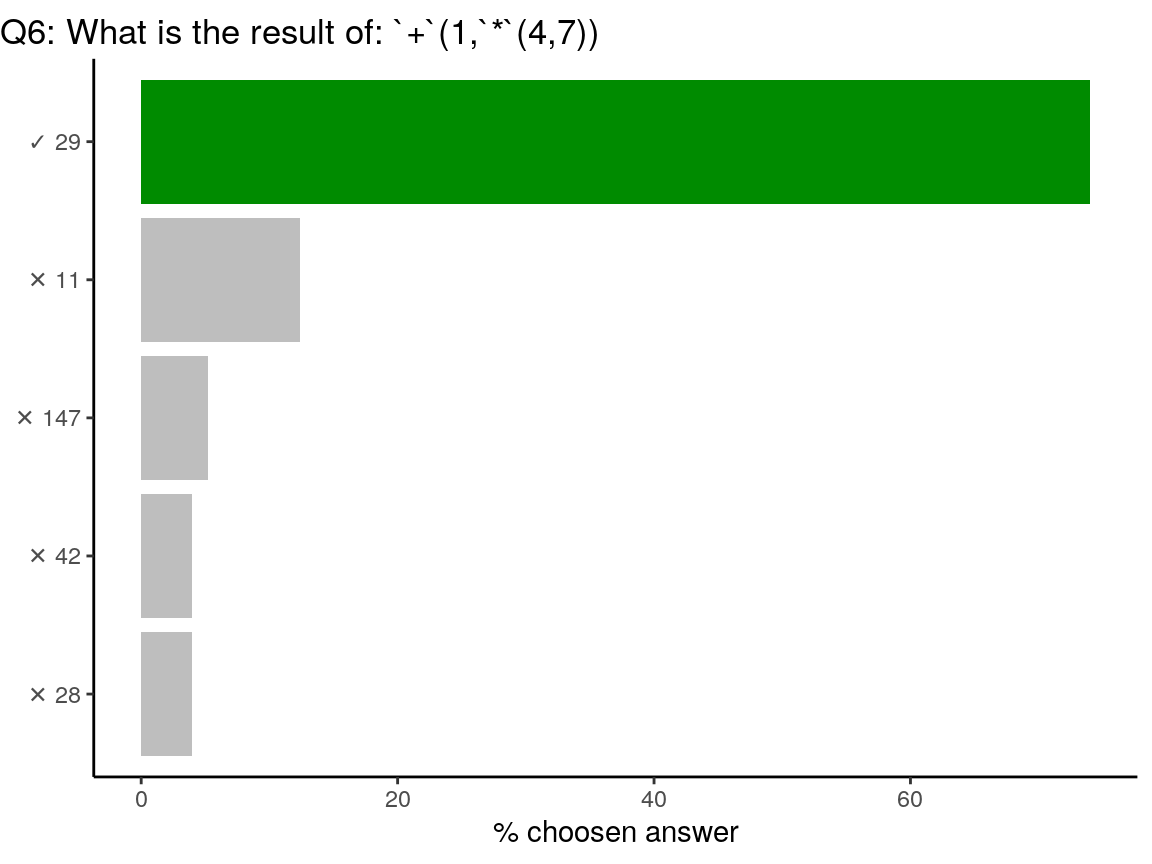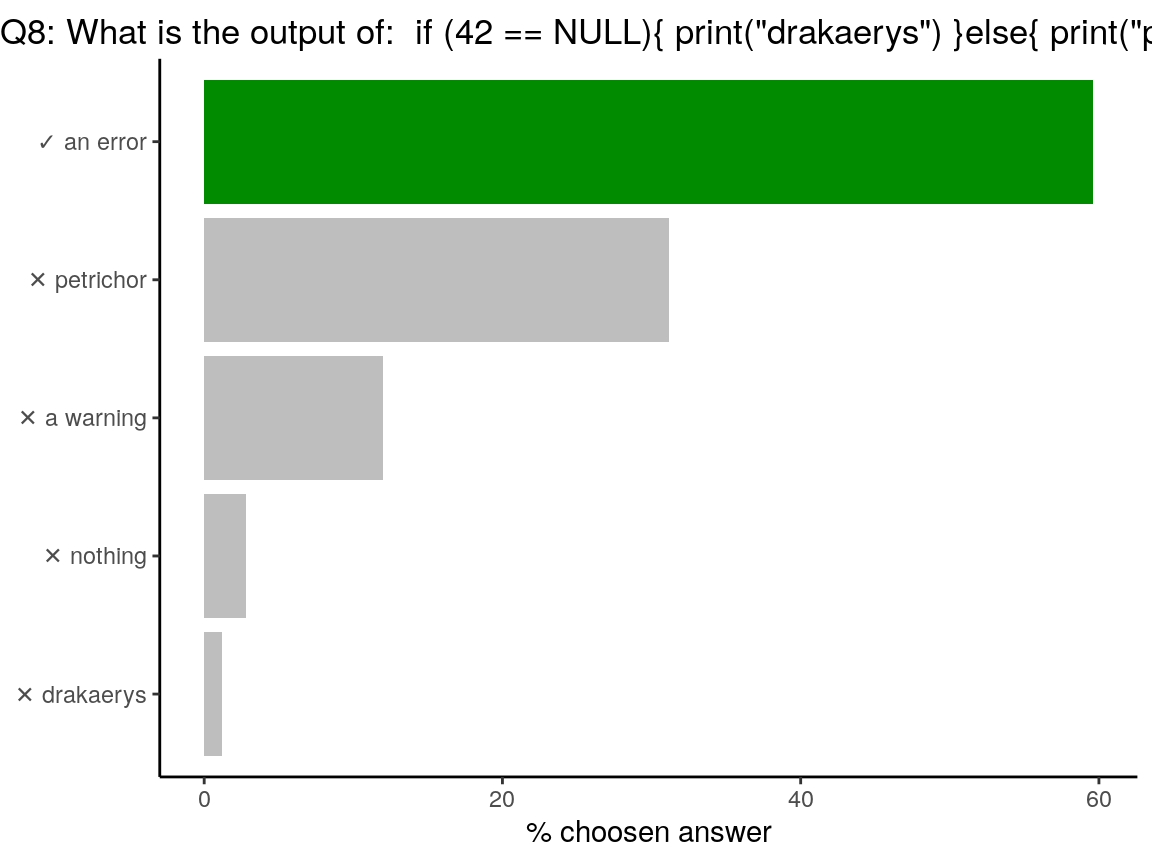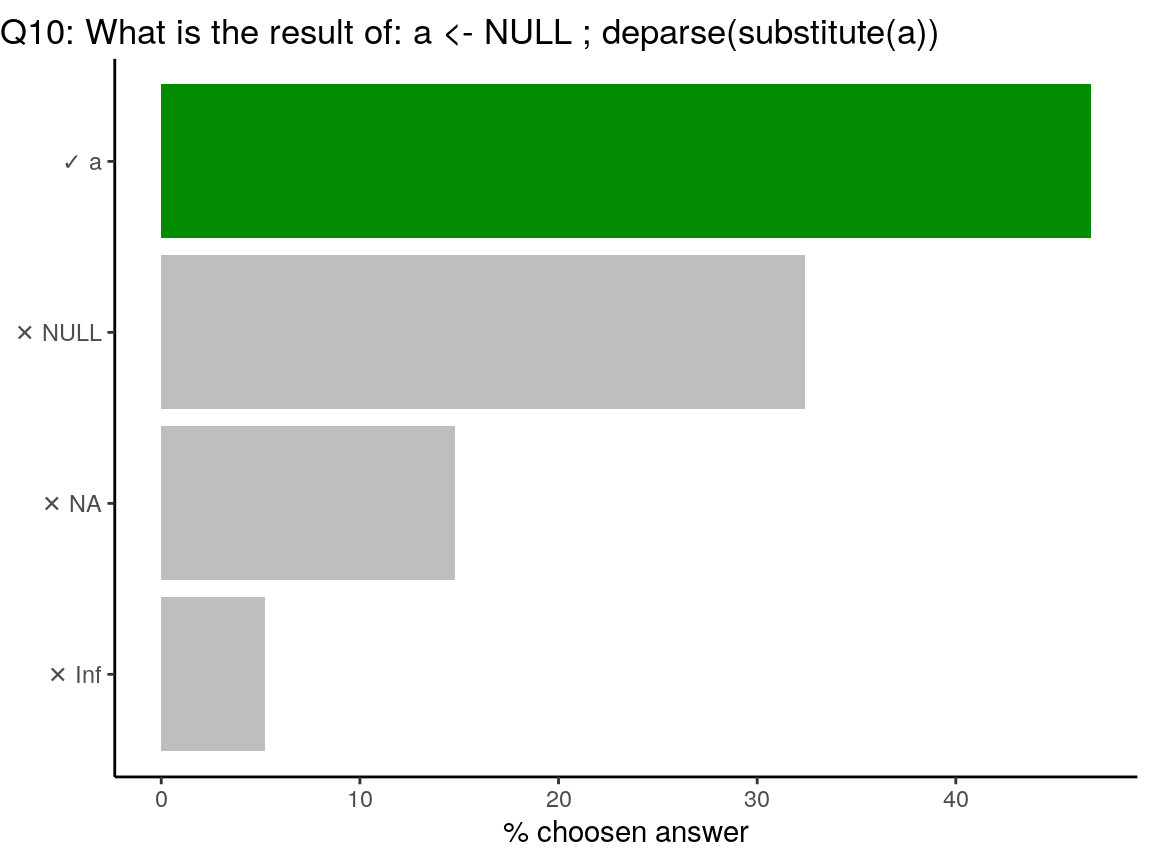# useR!2019 Quizz: Test your knowledge of base R and ThinkR

July 22, 2019
By

Want to share your content on R-bloggers? click here if you have a blog, or here if you don't.

At useR!2019 in Toulouse, ThinkR opened a quizz allowing to win a pipe knight. About a hundred of respondents won this Playmobil. In this blog post, we review the questions and respondents answers. We’ll see that the crowd as almost always right, but they do not know who the real R oracle is…

The quizz showed some difficulties and tricks of the base R language. This does not reflect your capacity to be a good R-programmer at all. This is only a game with tricky code that you surely do not want to write this way, but which is allowed by R. If you have ever been confronted to unattended behaviour with `NULL` values, some questions may speak to you (Isn’t that right, Colin?). Personnally, even after having read the answers twice, I am not sure I can have 100% good…To win a pipe knight people were required to score above 75% on wednesday, and above 60% on thursday and friday. We reduced the limit as respondents seemed to like the challenge of responding without opening an R session. Good attitude !## Libraries

``````library(readr)
library(dplyr)
library(tidyr)
library(ggplot2)
library(stringr)
library(grid)
library(gridExtra)``````

## Clean questions and link with choices

The dataset is not really tidy. We have to extract questions and choices first, and then respondent answers separately.List choices with the associated question and identify the correct answers.

``````# Read dataset
questions <- read_delim("QuizResponses_and_13.csv", delim = ",", n_max = 1, col_names = TRUE)
question_choice <- gather(questions, "question", "choice") %>%
mutate(question = if_else(grepl("^X\\d", question), NA_character_, question)) %>%
fill(question) %>%
mutate(question_id = str_extract(question, "(?<=^Q)(\\d+)(?=:)") %>% as.numeric()) %>%
filter(choice != "Points") %>%
mutate(correct = grepl("✓", choice))
question_choice``````
``````## # A tibble: 87 x 4
##    question                           choice            question_id correct
##
##  1 Q2: Which proposition(s) return(s… ✕ round(0.5) == …           2 FALSE
##  2 Q2: Which proposition(s) return(s… ✓ round(0.5) == …           2 TRUE
##  3 Q2: Which proposition(s) return(s… ✕ round(0.5) == …           2 FALSE
##  4 Q2: Which proposition(s) return(s… ✕ round(0.5) == …           2 FALSE
##  5 "Q3: What happens if:  dplyr <- \… ✕ Nothing                   3 FALSE
##  6 "Q3: What happens if:  dplyr <- \… ✓ it loads dplyr            3 TRUE
##  7 "Q3: What happens if:  dplyr <- \… ✕ it loads data.…           3 FALSE
##  8 "Q3: What happens if:  dplyr <- \… ✕ it returns an …           3 FALSE
##  9 "Q3: What happens if:  dplyr <- \… ✕ it loads both …           3 FALSE
## 10 Q4: What is the name of the Think… ✓ golem                     4 TRUE
## # … with 77 more rows``````

Let’s tidy the respondent answers dataset and join it with the table of questions and choices.

``````dataset <- read_delim("QuizResponses_and_13.csv", skip = 1, delim = ",")
rename(ID = X1) %>%
left_join(question_choice, by = "choice") %>%
arrange(question_id, choice)
``````## # A tibble: 28,000 x 6
##           ID choice           answer question           question_id correct
##
##  1   1.09e10 ✓ round(0.5) ==…    Q2: Which proposi…           2 TRUE
##  2   1.09e10 ✓ round(0.5) ==…    Q2: Which proposi…           2 TRUE
##  3   1.09e10 ✓ round(0.5) ==…    Q2: Which proposi…           2 TRUE
##  4   1.09e10 ✓ round(0.5) ==…    Q2: Which proposi…           2 TRUE
##  5   1.09e10 ✓ round(0.5) ==…    Q2: Which proposi…           2 TRUE
##  6   1.09e10 ✓ round(0.5) ==…    Q2: Which proposi…           2 TRUE
##  7   1.09e10 ✓ round(0.5) ==…    Q2: Which proposi…           2 TRUE
##  8   1.09e10 ✓ round(0.5) ==… 1      Q2: Which proposi…           2 TRUE
##  9   1.09e10 ✓ round(0.5) ==… 1      Q2: Which proposi…           2 TRUE
## 10   1.09e10 ✓ round(0.5) ==…    Q2: Which proposi…           2 TRUE
## # … with 27,990 more rows``````

``````total_correct <- sum(question_choice\$correct)
group_by(ID) %>%
summarise(points = sum(as.numeric(answer), na.rm = TRUE)) %>%
mutate(pc = 100 * points/total_correct) %>%
arrange(desc(pc))``````

Total number of respondents: 250.
Median percentage of correct answers: 54.
Number of participants with 100% correct answers: 3.

``````ggplot(pc_correct) +
geom_histogram(aes(pc), binwidth = 1, colour = thinkridentity::thinkr_cols("dark"), fill = thinkridentity::thinkr_cols("primary")) +
theme_classic() +
scale_x_continuous(limits = c(0, NA)) +
labs(x = "% correct", y = "Number of respondents",
title = "Distribution of percentage of correct answers")``````### Create function to build graph for each question

For each question, we will show the repartition of responses, ordered from most answered. The bar of the correct answers will be filled in green. There are also identified by a `✓` in the choices list.

``````#' Get question
#'
#' @param q_id id of the question
#'
#' @examples
#' get_question(2)
get_question <- function(q_id) {
question_choice %>%
filter(question_id == q_id) %>%
pull(question) %>%
unique()
}
#' Plot answers of a specific question
#'
#' @param q_id id of the question
#'
#' @examples
#' plot_question(2)
plot_question <- function(q_id) {
title <- get_question(q_id)
filter(question_id == q_id)
group_by(correct, choice) %>%
mutate(pc = 100 * nombre / n_respondents) %>%
ggplot(aes(reorder(choice, pc), pc, fill = correct)) +
geom_col() +
coord_flip() +
scale_fill_manual(values = c("TRUE" = "green4", "FALSE" = "grey"),
guide = FALSE) +
theme_classic() +
labs(y = "% choosen answer", x = NULL, title = NULL)
title.grob <- textGrob(
label = title,
x = unit(0, "lines"),
y = unit(0, "lines"),
hjust = 0, vjust = 0,
gp = gpar(fontsize = 13)
)
p1 <- arrangeGrob(p, top = title.grob)
grid.arrange(p1)
}``````

I could have write a loop for all figures, but I wanted to add some comments for some specific questions. So, here we go !
Question 1 was the email of respondents. This question is not part of the dataset.`?round` Details: Note that for rounding off a 5, the IEC 60559 standard (see also ‘IEEE 754’) is expected to be used, ‘go to the even digit’. Therefore round(0.5) is 0 and round(-1.5) is -2. However, this is dependent on OS services and on representation error (since e.g. 0.15 is not represented exactly, the rounding rule applies to the represented number and not to the printed number, and so round(0.15, 1) could be either 0.1 or 0.2).Code of the question

``````dplyr <- "data.table"
library(dplyr)``````

`library` is a r-base function doing non-standard evaluation (NSE). In the code above, `library` does not evaluate the R object named `dplyr`, but uses the symbol. Note that you can load {data.table} with `library("data.table")` for standard evaluation or use `library(dplyr, character.only = TRUE)` in this case. Who said “confusing”?

``library(dplyr, character.only = TRUE)``
``## data.table 1.12.2 using 2 threads (see ?getDTthreads).  Latest news: r-datatable.com``
``````##
## Attaching package: 'data.table'``````
``````## The following objects are masked from 'package:dplyr':
##
##     between, first, last``````Question was: Q4: What is the name of the ThinkR’s  package that provides a Framework for building robust shiny apps ?

{golem} is an opiniated framework for building production-grade shiny applications. Find out more about {golem} in its dedicated website. {remedy} provides addins to facilitate writing in markdown with RStudio. Obi-wan is legendary Jedi Master. Gerard is a random French name that you may find in the French {prenoms} database package.Q5: Among the members of the ThinkR team who is able to solve the rubik’s cube the fastest ?

Vincent owns dozens of Rubik’s cube at home. He trains every day! He beats us all…`+` and `*` are R functions. You can call them using ``` to be used with parenthesis like any other R function. It is also a way to call help on this function: `?`+``.

``````# Hence:
`+`(1, `*`(4, 7)) == (1 + (4 * 7))``````
``##  TRUE``The question was: Who does not have a tatoo? Colin tatoo’s are noticable. Vincent has one tatoo on the chest All other ThinkR members do not have any tatoo (for now…?).Code of the question

``````if (42 == NULL) {
print("drakaerys")
} else {
print("petrichor")
}
#> Error in if (42 == NULL) { : l'argument est de longueur nulle``````

In the `if` statement, the output should be of length `1`. Testing a value against `NULL` returns an object of length `0`.

``````# Test against NULL
(42 == NULL)``````
``## logical(0)``Code of the question

``````if (min(NULL) <= 42) {
print("drakaerys")
} else {
print("petrichor")
}``````
``## Warning in min(NULL): aucun argument trouvé pour min ; Inf est renvoyé``
``##  "petrichor"``

As said above, in the `if` statement, the output should be of length `1`. Contrary to the above question, `min(NULL)` is of length `1` as it returns `Inf`, with a warning. Hence `Inf` is not lower than `42`, and the test returns `petrichor`. Yes, R behaviour with `NULL` is tricky…

``min(NULL)``
``##  Inf``
``(min(NULL) <= 42)``
``##  FALSE``Code of the question

``````a <- NULL
deparse(substitute(a))``````
``##  "NULL"``

All is about evaluating or not evaluating an expression. `substitute(expr, env)` returns the parse tree for the (unevaluated) expression `expr`, substituting any variables bound in `env`. `deparse(expr, ...)` turns unevaluated expressions into character strings.

``````a <- NULL
# substitute
substitute(a)``````
``## NULL``
``substitute(a + 1, list(a = 2))``
``## 2 + 1``
``````# deparse
deparse(a)``````
``##  "NULL"``
``deparse(a + 1)``
``##  "numeric(0)"``
``````# deparse + substitute
deparse(substitute(a))``````
``##  "NULL"``
``deparse(substitute(a + 1))``
``##  "NULL + 1"``
``deparse(substitute(a + 1, list(a = 2)))``
``##  "2 + 1"``Code of the question

``````v <- c(1, NA, 3, 42, NaN)
# rlang::is_null is a wrapper around is.null
is.null(v) == rlang::is_null(v)``````
``##  TRUE``
``````# NA and NaN return TRUE with is.na()
sum(is.na(v)) == 1``````
``##  FALSE``
``````# is.na is vectorised and tests each value of `v`
# is.null tests the entire vector `v` and returns a unique value
length(is.na(v)) == length(is.null(v))``````
``##  FALSE``
``````# sum of values of a vector containing NA returns NA
# This tests returns NA
sum(v) == 46``````
``##  NA``Code of the question

``````b <- NULL
a <- 4 -> b
a * b``````
``##  16``

You can assign in both direction `<-` or `->`. Thus, value of `b` is changed in the second line of code.Code of the question

``````plop <- function(a, b, c, d) {
message("a =", a)
message("b =", b)
message("c =", c)
message("d =", d)
c
}
library(magrittr)
3 %>% plop(b = 1, 2, ., a = 4)``````
``##  2``

In a function, parameters are attributed in the order, except when they are explicitely named. In this case, `a` and `b` are named. The two remaining are attributed in the order: `c = 2` and `d = . = 3` (thanks to the `%>%`). Function `plop` returns `c`, hence `2`.Sorry Hadley… There was an indice in the question: `????`. This Easter Egg was introduced in August 14, 2008 by Duncan Murdoch: https://github.com/wch/r-source/commit/34b3998c928fbf50e24ab0e33c7d72ab8c944330

``????plop``
``````## Contacting Delphi...the oracle is unavailable.
## We apologize for any inconvenience.``````My presentation named “The “Rmd first” method: when projects start with documentation” is available on my github repository for presentations. It is augmented with a blog article ““Rmd first”: when projects start with documentation” on this Rtask Website. Also, that’s true I love R, I sometimes have fun with {sf} and of course, Lambert rules !Type (or class) of `NA` is logical, like `TRUE` or `FALSE`. In some cases, you may want to use `NA` in another type, like when using `if_else` or `case_when` in {dplyr}. In this case, you can use `NA_character_`, `NA_real_` or `NA_integer_`.

``class(NA)``
``##  "logical"``
``class(NA_character_)``
``##  "character"``
``class(NA_real_)``
``##  "numeric"``
``class(NA_integer_)``
``##  "integer"``In R, everything is a vector. `as.vector` does not transform the object into a one-dimension object. In the case of a data.frame, you can use `unlist` to transform as a 1D object.

``````# Original iris
data("iris")
class(iris)``````
``##  "data.frame"``
``dim(iris)``
``##  150   5``
``````# as.vector
iris2 <- as.vector(iris)
class(iris2)``````
``##  "data.frame"``
``dim(iris2)``
``##  150   5``
``````# As 1D with unlist
iris3 <- unlist(iris)
class(iris3) # factors are transformed as numeric``````
``##  "numeric"``
``dim(iris3)``
``## NULL``
``length(iris3)``
``##  750``{dplyr} provides a flexible grammar of data manipulation. Thus no graphics.
{rlang} provides tools to work with core language features of R and the tidyverse. Thus no graphics directly.
{lattice}: The lattice add-on package is an implementation of Trellis graphics for R.
{ggplot2} creates Elegant Data Visualisations Using the Grammar of Graphics.
{plotly} creates Interactive Web Graphics via ‘plotly.js’Code of the question

``````vec <- factor(c(3, 1, 11, 7))
mean(as.numeric(vec))
#>  2.5
# check the reason(s) why ?``````

factors are a specific type in R. Factors are stored as `integer` reflecting the order of the objects, and `levels`, but the class is `factor`. `unclass` removes the class `factor` to get the stored type of objects (ranks as integer).

``````vec <- factor(c(3, 1, 11, 7))
vec``````
``````##  3  1  11 7
## Levels: 1 3 7 11``````
``````# class
class(vec)``````
``##  "factor"``
``````# unclass
unclass(vec)``````
``````##  2 1 4 3
## attr(,"levels")
##  "1"  "3"  "7"  "11"``````
``````# test of unclass
setequal(unclass(vec), c(2, 1, 4, 3))``````
``##  TRUE``
``````# storage mode of the object
typeof(vec)``````
``##  "integer"``
``````# mean as none sense on factors
mean(vec)``````
``##  NA``
``````# as numeric, returns the ranks
as.numeric(vec)``````
``##  2 1 4 3``
``````# answer is the mean of the ranks
mean(as.numeric(vec))``````
``##  2.5``
``````# Let's see with letters?
vec2 <- factor(c("a", "b", "d", "c"))
class(vec2)``````
``##  "factor"``
``typeof(vec2)``
``##  "integer"``
``mean(vec2)``
``##  NA``
``mean(as.numeric(vec2))``
``##  2.5``‘Rmd first’ could have been my motto, considering the title of my useR!2019 presentation, but the one embroidered on my T-shirt was “I knight with {rmarkdown}”. For the other mottos, I let you find out…The post useR!2019 Quizz: Test your knowledge of base R and ThinkR appeared first on (en) The R Task Force.

R-bloggers.com offers daily e-mail updates about R news and tutorials about learning R and many other topics. Click here if you're looking to post or find an R/data-science job.
Want to share your content on R-bloggers? click here if you have a blog, or here if you don't.